Open in App
Not now

# Class 9 NCERT Solutions- Chapter 8 Quadrilaterals – Exercise 8.2

• Difficulty Level : Easy
• Last Updated : 13 Jan, 2021

### Question 1. ABCD is a quadrilateral in which P, Q, R, and S are mid-points of the sides AB, BC, CD, and DA (see Fig 8.29). AC is a diagonal. Show that:

(i) SR || AC and SR = ½ AC

(ii) PQ = SR

(iii) PQRS is a parallelogram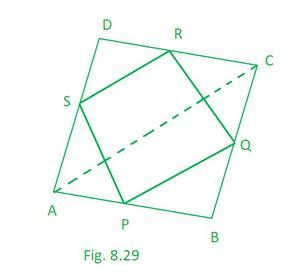Solution:

Given that, P, Q, R and S are the mid points of quadrilateral ABCD

Theorem 8.9: The line segment joining the mid-points of two sides of a triangle is parallel to the third side.

(i) So here, taking ∆ACD

we can see S and R are the mid points of side AD and DC respectively.    [Given]

Hence, SR || AC and SR = ½ AC (NCERT Theorem 8.9)……………………….(1)

(ii) So here, taking ∆ACB

we can see P and Q are the mid points of side AB and BC respectively.    [Given]

Hence, PQ || AC and PQ = ½ AC (NCERT Theorem 8.9)…………………………(2)

From (1) and (2) we can say,

PQ = SR

(iii) so from (i) and (ii) we can say that

PQ || AC and SR || AC

so, PQ || SR and PQ = SR

If each pair of opposite sides of a quadrilateral is equal, then it is a parallelogram.

Hence, PQRS is a parallelogram.

### Question 2. ABCD is a rhombus and P, Q, R and S are the mid-points of the sides AB, BC, CD and DA respectively. Show that the quadrilateral PQRS is a rectangle.

Solution:

Given that, P, Q, R and S are the mid points of Rhombus ABCD.

Theorem 8.9: The line segment joining the mid-points of two sides of a triangle is parallel to the third side.

Construction: Join AC and BD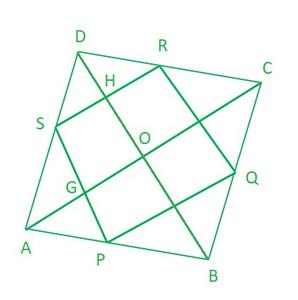So here, taking ∆ABD

We can see P and S are the mid points of side AB and AD respectively.    [Given]

Hence, PS || BD and PS = ½ BD (NCERT Theorem 8.9)……………………….(1)

Similarly, is we take ∆CBD

We can see R and Q are the mid points of side CD and CB respectively.    [Given]

Hence, RQ || BD and RQ = ½ BD (NCERT Theorem 8.9)……………………….(2)

So from (1) and (2), we conclude that

PS || RQ and PS = RQ

If each pair of opposite sides of a quadrilateral is equal, then it is a parallelogram.

Hence, PQRS is a parallelogram.

Now in ∆ACD

We can see S and R are the mid points of side AD and CD respectively.    [Given]

Hence, SR || AC and RS = ½ AC (NCERT Theorem 8.9)

from (2) RQ || BD and RQ = ½ BD (NCERT Theorem 8.9)

Hence, OGSH is a parallelogram.

∠HOG = 90° (Diagonal of rhombus intersect at 90°)

So ∠HSG = 90° (opposite angle of a parallelogram are equal)

As, PQRS is a parallelogram having vertices angles equal to 90°.

Hence, PQRS is a Rectangle.

### Question 3. ABCD is a rectangle and P, Q, R, and S are mid-points of the sides AB, BC, CD, and DA respectively. Show that the quadrilateral PQRS is a rhombus.

Solution:

Given that, P, Q, R and S are the mid points of Rectangle ABCD.

Construction: Join AC

Theorem 8.9 : The line segment joining the mid-points of two sides of a triangle is parallel to the third side.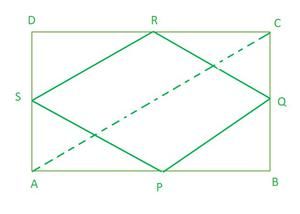So here, taking ∆ACD

we can see S and R are the mid points of side AD and DC respectively.    [Given]

Hence, SR || AC and SR = ½ AC (NCERT Theorem 8.9)……………………….(1)

Now, taking ∆ACB

we can see P and Q are the mid points of side AB and BC respectively.    [Given]

Hence, PQ || AC and PQ = ½ AC (NCERT Theorem 8.9)…………………………(2)

So from (1) and (2), we conclude that

SR || PQ and SR = PQ

Hence, PQRS is a parallelogram. (If each pair of opposite sides of a quadrilateral is equal, then it is a parallelogram)

Now in ∆QBP and ∆QCR

QC = QB (Q is the mid point of BC)

RC = PB (opposite sides are equal, hence half length is also equal)

∠QCR = ∠QBP (Each 90°)

∆QBP ≅ ∆QCR (By SAS congruency)

QR = QP (By C.P.C.T.)

As PQRS is a parallelogram and having adjacent sides equal

Hence, PQRS is a rhombus

### Question 4. ABCD is a trapezium in which AB || DC, BD is a diagonal and E is the mid-point of AD. A line is drawn through E parallel to AB intersecting BC at F (see Fig. 8.30). Show that F is the mid-point of BC.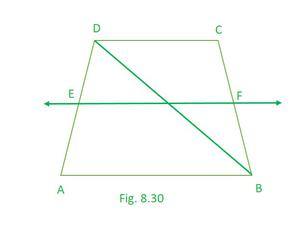Solution:

Let O be the point of intersection of lines BD and EF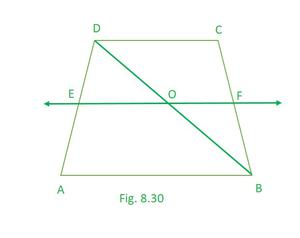Theorem 8.10 : The line drawn through the mid-point of one side of a triangle, parallel to another side bisects the third side.

we can see S is the mid point of side AD and ED || AB   [Given]

Hence, OD = ½ BD ………..(NCERT Theorem 8.10)

Now, taking ∆BCD

we can see O is the mid point of side BD and OF || AB   [Proved and Given]

Hence, CF = ½ BC…….. (NCERT Theorem 8.10)

Hence proved!!

### Question 5. In a parallelogram ABCD, E and F are the mid-points of sides AB and CD respectively (see Fig. 8.31). Show that the line segments AF and EC trisect the diagonal BD.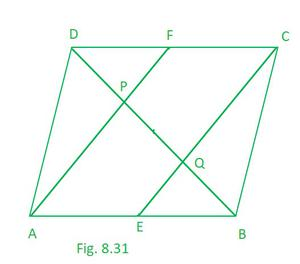Solution:

Theorem 8.10 : The line drawn through the mid-point of one side of a triangle, parallel to another side bisects the third side.

Given, E and F are the mid points of side AB and CD of parallelogram ABCD.

As, AB || CD and AB = CD  (opposite sides of parallelogram)………..(1)

AE = CF  (halves of opposite sides of parallelogram)………………………(2)

from (1) and (2)

AECF is a parallelogram

Hence, AF || EC

Now taking ∆APB

we can see E is the mid point of side AB and EF || AP   [Given and proved]

Hence, BQ = PQ………..(NCERT Theorem 8.10)………………….(1)

Now taking ∆CQD

we can see F is the mid point of side CD and CQ || FP   [Given and proved]

Hene, DP = PQ………..(NCERT Theorem 8.10)………………..(2)

From (1) and (2) we conclude that,

BQ = PQ = DQ

Hence, we can say that line segments AF and EC trisect the diagonal BD

### Question 6. Show that the line segments joining the mid-points of the opposite sides of a quadrilateral bisect each other.

Solution:

Theorem 8.9 : The line segment joining the mid-points of two sides of a triangle is parallel to the third side.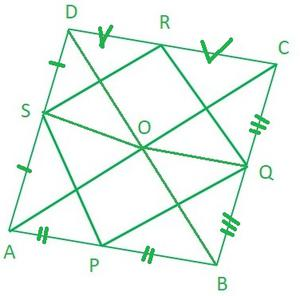So here, taking ∆ACD

we can see S and R are the mid points of side AD and DC respectively.    [Given]

Hence, SR || AC and SR = ½ AC (NCERT Theorem 8.9)……………………….(1)

Now, taking ∆ACB

we can see P and Q are the mid points of side AB and BC respectively.    [Given]

Hence, PQ || AC and PQ = ½ AC (NCERT Theorem 8.9)…………………………(2)

So from (1) and (2), we conclude that

SR || PQ and SR = PQ

Hence, PQRS is a parallelogram. (If each pair of opposite sides of a quadrilateral is equal, then it is a parallelogram)

And since the diagonal of parallelogram bisects each other

so, QS and PR bisects each other.

### Question 7. ABC is a triangle right angled at C. A line through the mid-point M of hypotenuse AB and parallel to BC intersects AC at D. Show that

(i) D is the mid-point of AC

(ii) MD ⊥ AC

(iii) CM = MA = ½ AB

Solution:

Theorem 8.10 : The line drawn through the mid-point of one side of a triangle, parallel to another side bisects the third side.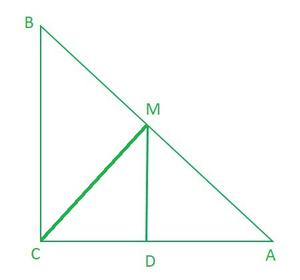(i) while taking ∆ABC

we can see M is the mid point of side AB and DM || BC   [Given]

This implies, DC= AD ……….. (NCERT Theorem 8.10)

Hence, D is the mid-point of AC.

(ii) As we know MD || BC and AC is transversal

This implies, ∠ACB = ∠ADM = 90°

Hence, MD ⊥AC

AD = CD (D is the mid point of AC (Proved))

∠CDM = ∠ADM (proved, MD ⊥AC)

DM = DM (common)

∆ADM ≅ ∆CDM (By SAS congruency)

CM = AM (By C.P.C.T.)

CM = AM = ½ AB (M is the mid point of AB)

My Personal Notes arrow_drop_up
Related Articles